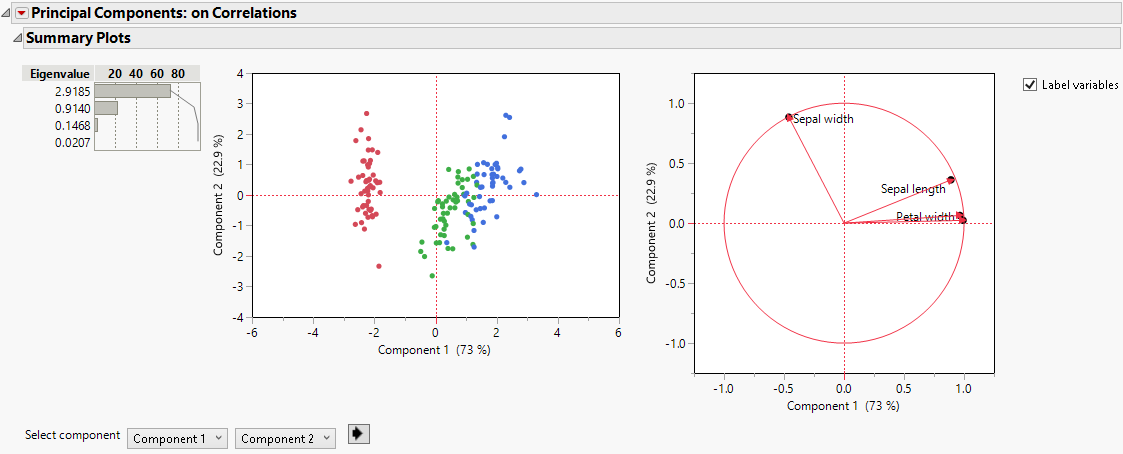Multivariate Methods > Principal Components
Publication date: 08/13/2020

# Principal Components

##### Reduce the Dimensionality of Your Data

The purpose of principal component analysis is to derive a small number of independent linear combinations (principal components) of a set of measured variables that capture as much of the variability in the original variables as possible. Principal component analysis is a dimension-reduction technique, as well as an exploratory data analysis tool. Principal component analysis is also useful for constructing predictive models, as in principal components analysis regression (also known as PCA regression or PCR).

For data with a very large number of variables, the Principal Components platform provides an estimation method called the Wide method. The Wide method enables you to calculate principal components in short computing times. These principal components can then be used in PCA regression.

For data that contain mostly zeros, also called sparse data, the Principal Components platform provides the Sparse estimation method. Similar to the Wide method, the Sparse method calculates principal components in short computing times. Unlike the Wide method, the Sparse method calculates a fixed, user-defined number of principal components rather than the full set.

The Principal Components platform also supports factor analysis. JMP offers several types of orthogonal and oblique factor analysis-style rotations to help interpret the extracted components. For factor analysis, see Factor Analysis.

Figure 4.1 Example of Principal Components